# Potential energy facts for kids

Kids Encyclopedia Facts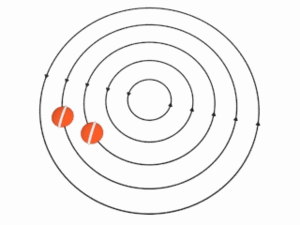The self-gravitational involution of a mass can be visualized as a series of concentric shells. The higher a shell, the lower its rotational frequency. If we extend the series of concentric shells to infinity, then the outermost shell's rotational frequency will be zero, so that the shell will have the lowest (i.e., zero) actual energy (nonzero‑frequency angular momentum) and the highest (i.e., zero) potential energy (zero‑frequency angular momentum).

A potential energy is the energy that an object has because of its position on a gradient of potential energy called a potential field, or just a potential.

• An actual energy (E = hf) is a nonzero‑frequency angular momentum.
• A potential energy is the zero‑frequency angular momentum stored in a potential flow of the vacuum.

The potential fields are irrotationally radial ("electric") fluxes of the vacuum and divide into two classes:

• The gravitoelectric fields;
• The electric fields.

The potential energy is negative. It is not a mere convention but a consequence of conservation of energy in the zero-energy universe—as an object descends into a potential field, its potential energy becomes more negative, while its actual energy becomes more positive.

In accordance with the "minimum total potential energy principle", the universe's matter flows towards the minimum total potential energy. This cosmic flow is time.

## Simple examples

Bringing a rock uphill increases (i.e., makes less negative) its gravitoelectric potential energy. Stretching a rubber band increases its elastic potential energy, which is a form of the electric potential energy. A mixture of a fuel and an oxidant has a chemical potential energy, which is another form of the electric potential energy. Batteries too have chemical potential energy.

## Gravitational potential energy

Gravitational potential energy is experienced by an object when height and mass is a factor in the system. Gravitational potential energy causes objects to move towards each other. If an object is lifted a certain distance from the surface from the Earth, the force experienced is caused by weight and height. Work is defined as force over a distance, and work is another word for energy. This means Gl Potential Energy is equal to: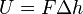$U = F \Delta h$
where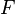$F$ is the force of gravity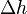$\Delta h$ is the change in height

or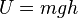$U = mgh$

Total work done by Gravitational Potential Energy in a moving object from position 1 to position 2 can be found by: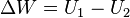$\Delta W = U_1-U_2$

or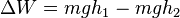$\Delta W = mgh_1-mgh_2$
where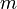$m$ is the mass of the object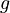$g$ is the acceleration caused by gravity (constant)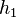$h_1$ is the first position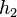$h_2$ is the second position

## Electric potential energy

Electric potential energy is experienced by charges both different and alike, as they repel or attract each other. Charges can either be positive (+) or negative (-), where opposite charges attract and similar charges repel. If two charges were placed a certain distance away from each other, the potential energy stored between the charges can be calculated by: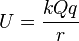$U = \frac{kQq}{r}$
where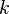$k$ is 1/4πє (for air or vacuum it is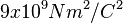$9 x 10^9 N m^2/C^2$)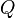$Q$ is the first charge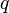$q$ is the second charge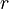$r$ is the distance apart

## Elastic potential energy

Elastic potential energy is experienced when a rubbery material is pulled away or pushed together. The amount of potential energy the material has depends on the distance pulled or pushed. The longer the distance pushed, the greater the elastic potential energy the material has. If a material is pulled or pushed, the potential energy can be calculated by: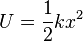$U = \frac{1}{2}kx^2$
where$k$ is the spring force constant (how well the material stretches or compresses)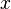$x$ is the distance the material moved from its original position

## Images for kidsPotential energy Facts for Kids. Kiddle Encyclopedia.